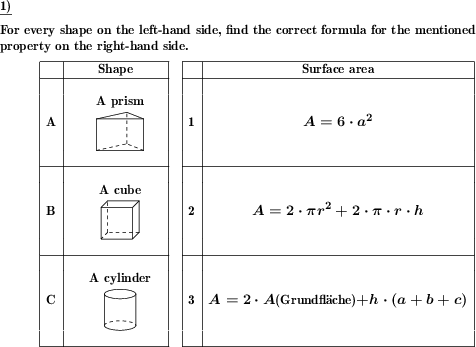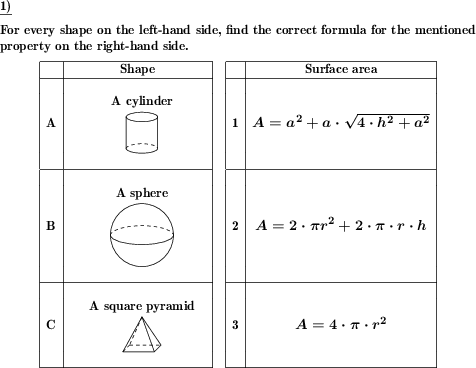Custom math worksheets at your fingertips# Details for problem "Shapes 3d: Match shape and volume or surface formula"

Quickname: 6031

Elementary School, Primary School, Junior High School, Middle School, High School, Senior High School.

## Summary

Match named or shown three-dimensional geometric shapes to the correct formula for volume or surface area.

## Examples## Description

A series three-dimensional geometric shapes is shown in text form or as a drawing in a table. Another table shows the formulas for either the volume or the surface areas of each shape, in a random order. The task is to match each shape to the correct formula.

The number of shapes presented is selectable. It can be configured whether the shapes are identified by their names or by an image or both. The type of formula that has to be matched can be chosen.

In the left table with the list of shapes, the lines are numbered with letters. In the right table, they are numbered with numbers. So each letter has to be matched with the correct numbers.

For every shape, it is selectable whether it will appear in the problem statement. Possible shapes are:

- cube/cuboid
- prism
- (square) pyramid
- (regular) tetrahedron
- cylinder
- cone
- sphere

Download free printable worksheets for this math problem here. The worksheet contains the problems only, the solution sheet includes the answers. Just click on the respective link.

•Worksheet 1Solution sheet with answers
•Worksheet 2Solution sheet with answers
•Worksheet 3Solution sheet with answers

If you can not see the solution sheets for download, they may be filtered out by an ad blocker that you may have installed. If this is the case, please allow ads for this page and reload the page. The solution sheets will then reappear.

• Do these sample worksheets do not really fit?
• Do you need more math worksheets, with a different level of difficulty?
• Would you like to combine different problems on a worksheet and adjust them to your needs?
• As a teacher, you can put together your own worksheets using the automatically generated math problems provided.
With a free initial credit, you can start creating your own math worksheets in a few minutes.

You can try it for free! Register here, to create custom worksheets now!

## Customization options for this problem

Parameter
Possible values
Number of problem
2, 3, 4, 5, 6
surface area, volume
Given
name, image, name and image
Cube/cuboid
Yes, No
Prism
Yes, No
Tetrahedron
Yes, No
Pyramid
Yes, No
Cylinder
Yes, No
Sphere
Yes, No
Cone
Yes, No

## Similar problems

Remark
Description
Involving calculation of actual value: Calculate surface area and volume for given geometric shape, or state formulas
For three-dimensional geometric shapes, state the formula for the volume and/or surface area, or calculate these values.Deutsche Version dieser Aufgabe
These informational pages with samples describe math problems that can be combined on custom math worksheets with solutions for home and K-12 school use.
Deutsche Seiten
×